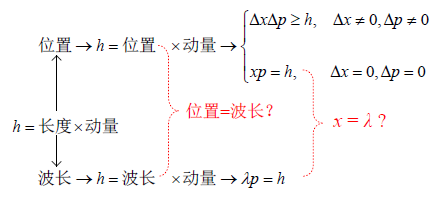﻿ 试析不确定性原理与物质波概念之间的矛盾

# 试析不确定性原理与物质波概念之间的矛盾An Analysis of the Contradiction between the Principle of Uncertainty and the Concept of Material Wave

Abstract: The relationships among the mass, energy and momentum of photon are introduced. It is pointed out that the wavelength and frequency of photon are auxiliary variables of no physical significance. The uncertainty principle and the concept of matter wave play a fundamental role in quantum mechanics. Both of them are all related to Planck’s constant. Based on the dimensional analysis method, their relationships with Planck’s constant are discussed. The results show that the uncertainty principle and the concept of matter wave are contradictory.

1. 引言

2. 光子质量、能量和动量三者之间的关系

$能量\equiv 质量×{\left(速度\right)}^{2}$ (1)

$E=M{c}^{\text{2}}$ (2)

$E=\frac{hc}{\lambda }=hv$ (3)

$1\text{\hspace{0.17em}}\text{J}=1\text{\hspace{0.17em}}\text{kg}\cdot {\left(\text{m}/\text{s}\right)}^{\text{2}}$ (4)

$M=\frac{h}{\lambda c}$ (5)

$动量\equiv 质量×速度$ (6)

$\frac{E}{c}=Mc$ (7)

$p=Mc$ (8)

$Mc=p=\frac{h}{\lambda }$ (9)

3. 光子的波长和频率是没有物理意义的辅助变量

4. 从普朗克常数的角度看不确定性原理和物质波概念之间的矛盾

$长度\left(的量纲\right)×速度\left(的量纲\right)×质量\left(的量纲\right)\equiv 普朗克常数h\left(的量纲\right)$ (10)

$长度×速度×质量\equiv h$ (11)

$长度×动量\equiv h$ (12)

$长度×质量×速度\ge h$ (13)

$长度×动量\ge h$ (14)

$\left(x+\Delta x\right)\cdot \left(p+\Delta p\right)=x\cdot p+x\cdot \Delta p+\Delta x\cdot p+\Delta x\cdot \Delta p$ (15)

$x\cdot p>x\cdot \Delta p>\Delta x\cdot p>\Delta x\cdot \Delta p$

$\left\{\begin{array}{l}x\cdot p\ge h\\ x\cdot \Delta p\ge h\\ \Delta x\cdot p\ge h\\ \Delta x\cdot \Delta p\ge h\end{array}\text{}\Delta x\ne 0,\Delta p\ne 0$ (16)

$\Delta x\cdot \Delta p\ge h$ (17)

$p+\Delta p=\left(m+\Delta m\right)\cdot \left(u+\Delta u\right)=m\cdot u+m\cdot \Delta u+\Delta m\cdot u+\Delta m\cdot \Delta u$ (18)

$\begin{array}{c}\left(x+\Delta x\right)\cdot \left(p+\Delta p\right)=\left(x+\Delta x\right)\cdot \left(m+\Delta m\right)\cdot \left(u+\Delta u\right)\\ =x\cdot m\cdot u+x\cdot m\cdot \Delta u+x\cdot \Delta m\cdot u+x\cdot \Delta m\cdot \Delta u\\ \text{\hspace{0.17em}}\text{\hspace{0.17em}}+\Delta x\cdot m\cdot u+\Delta x\cdot m\cdot \Delta u+\Delta x\cdot \Delta m\cdot u+\Delta x\cdot \Delta m\cdot \Delta u\end{array}$ (19)

$\left\{\begin{array}{l}x\cdot m\cdot u\ge h\\ x\cdot m\cdot \Delta u\ge h\\ x\cdot \Delta m\cdot u\ge h\\ x\cdot \Delta m\cdot \Delta u\ge h\\ \Delta x\cdot m\cdot u\ge h\\ \Delta x\cdot m\cdot \Delta u\ge h\\ \Delta x\cdot \Delta m\cdot u\ge h\\ \Delta x\cdot \Delta m\cdot \Delta u\ge h\end{array}\text{}\Delta x\ne 0,\Delta m\ne 0,\Delta u\ne 0$ (20)

$\Delta x\cdot \Delta m\cdot \Delta u\ge h$ (21)

$\left\{\begin{array}{l}x\cdot m\cdot u\ge h\\ x\cdot m\cdot \Delta u\ge h\\ \Delta x\cdot m\cdot u\ge h\\ \Delta x\cdot m\cdot \Delta u\ge h\end{array}\text{}\Delta x\ne 0,\Delta u\ne 0$ (22)

$\Delta x\cdot m\cdot \Delta u\ge h$ (23)

Δm = 0实际上引入了质量不变或者质量守恒的概念。但是不能因为质量守恒就认为质量没有不确定性，因为毕竟质量具体有多少也是测量出来的。所以广义的不确定性原理应该采用式(21)。

$\left(x+\Delta x\right)\cdot \left(p+\Delta p\right)=x\cdot p=h$ (24)

$x=\frac{h}{p}$ (25)

$p=\frac{h}{x}$ (26)

$\lambda =\frac{h}{p}$ (27)

$p=\frac{h}{\lambda }$ (28)

$\lambda p=h$ (29)Figure 1. Two relations corresponding to Planck’s constant h

$p=Mc$ (30)

$M=\frac{h}{\lambda c}$ (31)

$M=\frac{E}{{c}^{2}}$ (32)

$E=\frac{hc}{\lambda }=hv$ (33)

5. 结束语

“物质波以及波动现象在量子论里有较大的作用  (第46页)”，另一方面，不确定性原理“是量子力学的组成部分，同时也是它的天然而直接的结论  (第57页)”。如果剔掉物质波概念，再加上不确定性原理没有多少物理意义，量子力学理论的基础或将被动摇。

 王忆锋. 光速原理及其推论[J]. 现代物理, 2019, 9(5): 227-245.

 王忆锋. 论光子本性的公理化逻辑分析[J]. 云光技术, 2017, 49(3): 41-65.

 王忆锋. 论若干物理问题的量纲分析方法[J]. 云光技术, 2018, 50(2): 42-51.

 王忆锋. 基于量纲分析从物理和数学角度推导光速原理[J]. 现代物理, 2019, 9(5): 183-190.

 沈乃澂. 基本物理常数1998年国际推荐值[M]. 北京: 中国计量出版社, 2004.

 赵凯华, 罗蔚茵. 量子物理[M]. 北京: 高等教育出版社, 2001.

 犬石嘉雄, 滨川圭弘, 白藤纯嗣. 半导体物理[M]. 张志杰, 郗小林, 雷京贵, 等, 译. 周绍康, 校. 北京: 科学出版社, 1986.

 野村昭一郎. 量子力学入门[M]. 李彬, 黄东律, 康昌鹤, 等, 译. 北京: 高等教育出版社, 1985.

 D. S. 萨克森. 初等量子力学[M]. 苏耀中, 叶安祚, 译. 北京: 高等教育出版社, 1985.